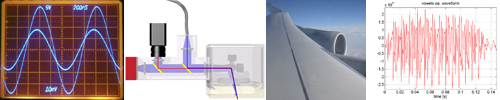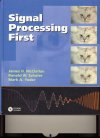## Mathematical Methods in Electrical Engineering

Dates for lectures and exercises, see RWTHOnline

### Small group exercises

There are 22 small group exercises offered. Further information via RWTHOnline.

Note: The small group exercises require registration. (The registration procedure coordinates the registrations for the different small group exercises of the 1st semester in the bachelor program Electrical Engineering, Information Technology and Computer Engineering, so that an overlap-free participation in the registered exercise groups is possible for each student according to the respective prioritization).

Due to the availability of more and more powerful computers and processor platforms, electrotechnical systems are increasingly realized in the form of “digital” processes. Examples are cell phones, DVB, DVD, CD, MP3, but also professional systems e.g. of medical imaging. This lecture complements the basic modules on analog components and networks with content on digital processes, some of which are implemented on computers under MATLAB. The focus is on mathematical methods for digital processing of audio, image and video signals.

The lecture is accompanied by an introduction to the programming language and development environment MATLAB, which is used to implement the lecture contents on computers.

### Contents of the lecture

1. Introduction: Computer Aided Signal Processing, Signals & Systems, Introduction to MATLAB
2. Sine signals, complex sine signals, Euler’s formula
3. Spectral representation of signals, Fourier series
4. Sampling, A/D and D/A conversion
5. Filtering: linear and time-invariant systems, convolution
6. Fourier transform, transfer function and spectrum
7. Polynomials: normal representation, linear factorization, zero diagrams, fundamental theorem of algebra
8. z-transform, system function and filter design
9. Scalar product, discrete Fourier transform (DFT), matrix representation
10. Linear systems of equations (Gaussian elimination, Cramer’s rule)

### Literature for the lectureJ. H. McClellan, R. W. Schafer, M. A. Yoder: Signal Processing First, Pearson Prentice-Hall, 2003

The lecture covers chapters 1-7 and chapter 13 in addition to basics from mathematics.

Teaching materials are accessible via the teaching and learning portal Moodle of RWTH Aachen University:

http://www.elearning.rwth-aachen.de

#### Resources

MMET-MATLAB-Primer_v2.2